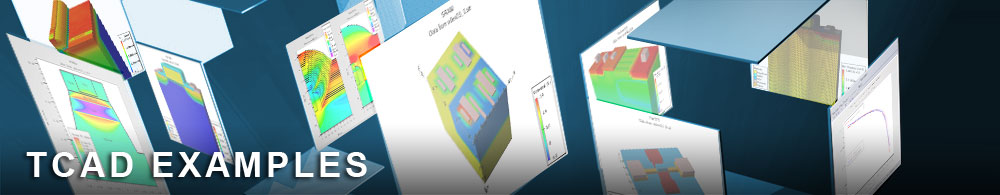Threshold Voltage Hysteresis of a Silicon nMOSFET

## mos2ex19.in : Threshold Voltage Hysteresis of a Silicon nMOSFET

Requires: S-Pisces
Minimum Versions: Atlas 5.28.1.R

This example shows how to simulate the finite charging and discharging times of interface traps without doing a transient simulation. The example demonstrates:

• How to use the sweep and save parameters of dbinternal.
• Set up a deck with interface traps extending into the oxide.
• Do a steady-state voltage ramp while simulating the temporal response of traps.
• Obtain and visualise the threshold voltage differences as a function of simulated ramp time.

The file mos2ex19.in starts dbinternal and instructs it to use mos2ex19_aux.in. The statement
sweep parameter=measurementtime type=power range="1.0, 1.0e-4, 9"
causes the deck to be run 9 times in succession with the value of measurement time varying between 1.0 and 1.0e-4 in a semi-decade decrease each time. The file mos2ex19_aux.in sets up a basic nMOSFET, and the statement
inttrap e.level=0.6 acceptor density=1.0e12 s.i degen=4 sign=1.0e-13 sigp=1.0e-14 x.min=0.6 x.max=0.9 heiman depth=0.002 hpoints=20
sets up a uniform distribution of acceptor-like traps along part of the Si/SiO2 interface. The areal density is 1e12 /cc and the depth is 0.6 eV below the Silicon conduction band. The HEIMAN flag causes the traps to have a uniform distribution into the interface to a depth of 2 nanometres, covered by a vertical interpolation mesh of 20 points. How this affects the capture and emission times of the carriers is explained in the manual. The solve statement parameter timespan is assigned a value from the current value of measurementtime, and supplies the overall time for the ramp to the HEIMAN model. The drain is biased to conduct and then the gate voltage is ramped from 0V to 3V. The trap occupancy is then calculated at each bias step according to the bias value and the elapsed time. At the end of the forward ramp, the gate voltage is ramped down to 0 V again, with the same value assigned to timespan.

The threshold voltages and their difference are calculated and are output to the file mos2ex19.dat in a format suitable for viewing by Tonyplot. This was specified in the statement
save type=sdb outfile=mos2ex19.dat
in mos2ex19.in. The log files for forward and reverse gate bias ramps are also output, and the ones plotted correspond to the last value of measurement time used (0.1 milliseconds).

The traps are initially empty and as the bias increases they start to fill with electrons. For a short measurement time the traps occupation fraction is small when the threshold gate voltage is exceeded and so there is no significant effect on the threhold voltage. At the end of the ramp the trap occupation is higher. A solve prev at this stage would cause the trap occupation probabilities to achieve their steady state values. Instead, the gate voltage ramp is reversed and the traps continue to charge until the gate voltage is reduced enough that carrier emission starts to dominate. The threshold voltage occurs while the traps still have a high occupation probability and contibute a negative interface charge. Consequently the threshold voltage is higher on the reverse ramp. This difference is plotted as a function of measurement time, and is more pronounced for shorter simulated measurement times. For longer measurement times the traps have time to respond to the bias changes and no hysteresis effects are seen. Typical Id-Vg curves and trap occupation probabilities versus Vg curves are shown for a measurement time of 0.1 milliseconds. They clearly demonstrate the hysteresis. Actual Vt values can also be plotted from mos2ex19.dat.

To load and run this example, select the Load button in DeckBuild > Examples. This will copy the input file and any support files to your current working directory. Select the Run button in DeckBuild to execute the example.